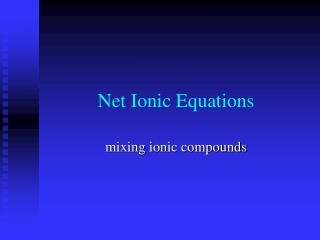DownloadDownload PresentationNet Ionic Equations

# Net Ionic Equations

Télécharger la présentation## Net Ionic Equations

- - - - - - - - - - - - - - - - - - - - - - - - - - - E N D - - - - - - - - - - - - - - - - - - - - - - - - - - -
##### Presentation Transcript

1. Net Ionic Equations mixing ionic compounds

2. Ionic interactions • When you dissolve 2 or more ionic compounds in water some parts of it may react together and precipitate out • Precipitate-solid falling out of solution • More times than not, most (if not all) of the ions do nothing • Net ionic equations are only concerned with the ions that do something

3. This goes back to ionic dissociation in water NaCl(s) + AgNO3(s)in water Na+(aq)+ Cl-(aq) + Ag+(aq) + NO3-(aq) Net ionic ignores everything that doesn’t do anything! AgCl(s) + Na+(aq)+ NO3-(aq) Ag+(aq) +Cl-(aq) AgCl(s) net ionic equation-- Here’s the trick, AgCl is insoluble

4. More net ionic equations • You normally write the reactants as aqueous (since you are dissolving them) • Mg(NO3)2(aq) + Na2CO3(aq) MgCO3(s) + 2NaNO3(aq) • Dissociate everything that is aqueous, not solid • Mg++ + 2NO3- + 2Na+ + CO3-- •  MgCO3(s) + 2 Na+ + 2NO3- • Now cancel out everything that is the same on both sides of the equation • These are called spectator ions The remaining part is the net ionic equation Mg++ + CO3--  MgCO3(s)

5. What if nothing precipitates out? • K2SO4(aq)+AgNO3(aq) KNO3(aq) + Ag2SO4(aq) • (by the way, this equation isn’t balanced) • 2 K+ + SO42- + Ag+ + NO3- • K+ + NO3- + 2 Ag+ + SO42- • Everything cancels out • There is no net ionic equation

6. Practice with equations that are not yet balanced • K2CO3(aq)+ Ca(NO3)2(aq) KNO3(aq) + CaCO3(s) • 2K+ + CO32- + Ca++ + 2 NO3- • CaCO3(s) +K+ + NO3- • Just ignore coefficients when canceling • Ca++ + CO32- CaCO3(s) • If it were unbalanced now you would balance it

7. Tip to make sure your answer is right • Make sure you have the same atoms on each side of the equation • If your final answer is something like • K+ + CO32- CaCO3(s) • It is wrong!!! • Atoms don’t spontaneously change into other atoms (without a nuclear reaction)

8. more • K2C2O4(aq)+ CuNO3(aq) KNO3(aq) + Cu2C2O4(s) • Pb(ClO3)4(aq)+ NiCl2(aq) Ni(ClO3)2(aq) + PbCl4(s)

9. Homework • Na3PO4 (aq) + Ba(NO3)2 (aq) •  NaNO3 (aq) + Ba3(PO4)2 (s)